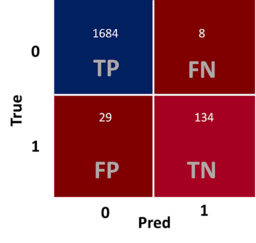# 【Python 機器學習】：混淆矩陣 – 驗證分類模型的瑞士刀## 使用 Python 產生混淆矩陣

from sklearn.metrics import confusion_matrix
cm=confusion_matrix(y_test,y_pred)
cm

• y_test (ground truth) 是資料當中測試資料的目標陣列
• y_pred (model prediction) 是模型根據X_test 去進行預測後的結果

## 查看y_test當中的標籤個數

y_test.value_counts()

## 將混淆矩陣畫出，加以解釋

import seaborn as sns
import matplotlib.pyplot as plt
plt.figure(figsize=(15,8))
sns.heatmap(cm,square=True,annot=True,fmt='d',linecolor='white',cmap='RdBu',linewidths=1.5,cbar=False)
plt.xlabel('Pred',fontsize=20)
plt.ylabel('True',fontsize=20)
plt.show()

### 四個方格

• (1) TP (True Postitive): 真實情況為class_0，預測也為class_0的個數有1684個
• (2) FN (False Negative): 真實情況為class_0，預測為class_1的個數有8個
• (3) FP (False Postitive): 真實情況為class_1，預測為class_0的數有29個
• (4) TN (True Negative): 真實情況為class_1，預測為class_1的個數有134個
• FP (又稱為偽陽性)就是統計學上的型一錯誤 (Type I Error)
• FN (又稱為偽陰性)就是統計學上的型二錯誤 (Type II Error)
• 小技巧: 上面竟然都說是錯誤了，當然都是 F 開頭

### 常用的指標

• (1) Accuracy(準確率)
• $$\frac{TP+TN}{TP+TN+FP+FN} = \frac{1684 + 134}{1855} = 0.98$$
• 準確率就是當他是 class_0(正例) 且預測為class_0(正例) 或是 class_1(負例) 且預測也為class_1(負例) 這兩種情況下都算是準確，因此根據上述公式即可算出準確率。
• 簡言之，準確率就是所有預測正確 (分子都是以T開頭) 的比例
• (2) Precision(精確率):
• $$\frac{TP}{TP + FP} = \frac{1684}{1684 + 29} = 0.98$$
• 精確率的意思是在預測出是class_0(正例)的情況下，真實情況也為class_0(正例)的比率
• 精確率就是所有預測正例 (分母都是以P結尾) 中，真實也是正例的比率
• (3) Recall(召回率):
• $$\frac{TP}{TP+FN} = \frac{1684}{1684+8} =0.99$$
• 精確率的意思是真實情況為 class_0 (正例) 的情況下，預測是 class_0 (正例) 的比率
• 精確率就是所有正例中，預測也為正例的比率
• (4) F1 Score:
• $$F1=\frac{2}{\frac{1}{Precision} + \frac{1}{Recall}} = \frac{2}{\frac{1}{0.98} + \frac{1}{0.99}} = 0.98$$

## 直接產出所有數值的報告

from sklearn.metrics import classification_report

print(classification_report(y_test,y_pred))

## 後記Grandma2